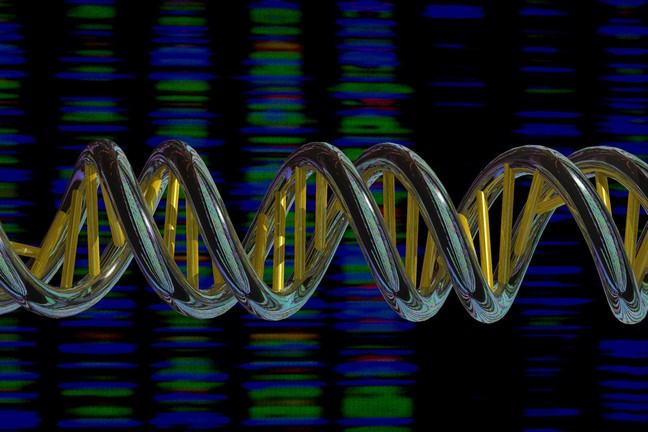2.10

## Wellcome Genome Campus Advanced Courses and Scientific ConferencesDNA double helix and sequence output

# Genomes can have different percentages of G and C bases/nucleotides

In this Step we will learn about the GC (guanine-ctytosine) content of a DNA and how to calculate it. This will be useful for later Steps.

One of the characteristics of genomes is the proportion of Guanines (G) and Cytosines (C) that their genomes have. Because G=C bonds are stronger than A=T bonds, differences in GC content can indicate differences in the properties of the DNA. For example, if we consider two “naked” DNA molecules (that is without any protein attached to them) of the same length but different GC content, it is expected that the molecules with higher GC content are more difficult to separate from each other because they are bonded together with greater “strength”. The physical properties of AT-rich and GC-rich sequences of DNA are therefore different.

To calculate the GC content of a genome or DNA molecule, we simply count the number of G and C and divide them by the total number of bases, that is G+C+A+T. For example, a 1,000 nucleotide long DNA molecule has 345 G and 370 C, the GC content is:

345 (G) + 370 (C) / 1000 (G+C+A+T) = 0.715


We can multiply this figure by 100 to make it a percentage

100 * 0.715 = 71.5 % GC


How can GC content be useful to learn more about gene features or genomic regions (smaller regions of the genome) if the same value of GC content applies to the whole genome?